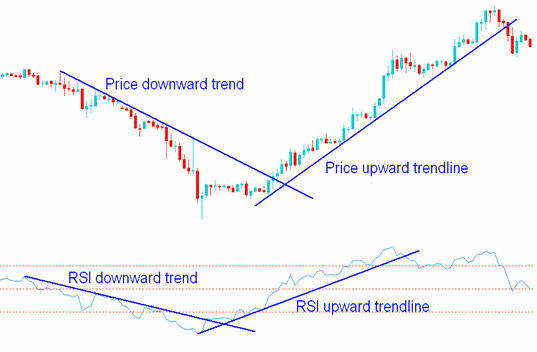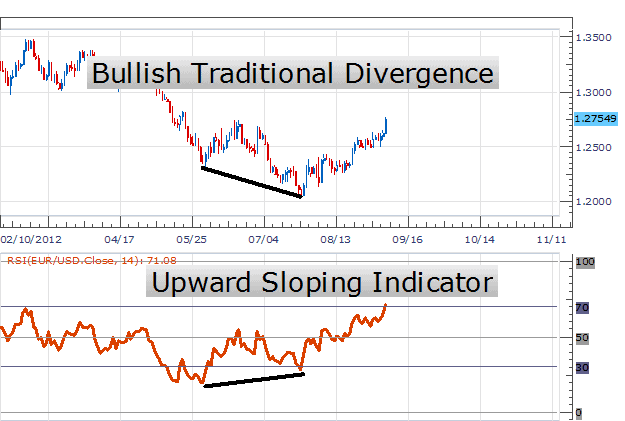Understanding the Basics of Relative Strength Index RSI in forex

What is Relative Strength Index?
In the year 1978, J. Welles Wilder, Junior introduced an oscillator called The Relative Strength Index RSI. It is mostly used by the investors who want to invest in the stock market as the index evaluates the price of a commodity relative to itself and compute the price action’s momentum. The rate of change or momentum indicator is referred by an oscillator. The values are mostly ranges from negative one to positive one or zero to hundred percent. It is precisely known as an internal strength index.How does the Relative Strength Index Works?
Mostly a period ranges between 5 and 25 days is ideal for the measurement of the RSI. It’s calculations for a given period of time are based upon the difference between the average of the closing value on up days and the average closing value on the down days. The values taken by this method are then plotted on vertical scale that ranges from zero to hundred.
The period allocated by Wilder was of 14 day Relative Strength Index. However, due to market’s positive characteristics the long or short periods have also acquired popularity.
The Relative Strength Index Equation
The value of the is calculated with the help of an equation
1
________________________________________
1 + U/D
In this equation:
U stands for the average of upward movement.
D stands for the average of downward movement.
This sort of calculation is used by the RSI Classic.
The difference between the RSI Classic formula and RSI Conventional Formula:
The real method of IQ Chart’s for demonstrating the indicator is the RSI, where as the RSI classic is the conventional computation of the usual Relative Strength Index indicator.
In IQ Chart’s RSI, U is calculated as the total of upward movement and D as the total of downward movement. On the contrary in classic RSI, U is calculated as an average number of up days/upward movement and D is calculated as a an average number of down days/downward movement.
How to Interpret the Relative Strength Index:
The interpretations of the Relative Strength Index purely depend upon the approach of investment and trading or the condition of the market. One can find different possible interpretations of the RSI.

• One of the interpretations could be looking for the formations of common chart, resistance lines like the head and shoulders in the Relative Strength Index itself, which indicates the prospective reversals that might not be indicated on the stock chart.

• Another interpretation could be that when RSI is in oversold area which is in between twenty to thirty then the buy indicators are triggered and if the overbought ranges in between seventy to eight, the signals for sell are triggered. The first condition refers that a market is showing a low trend; where as the other condition shows a high trend of the market.

• The average for the Relative Strength Index is fifty. With this value the trend of the market can be recognized that whether it’s bullish or bearish. The value above fifty is counted bullish where as the below fifty is counted bearish.

• Recognizing the Relative Strength Index divergence could be a good tool of interpretation. Having a keen eye on the movement of RSI, like when the prices are going down or up would be beneficial.